# Pulse Width Modulation PWM Pulse Width Modulation PWM

• Slides: 11
Download presentation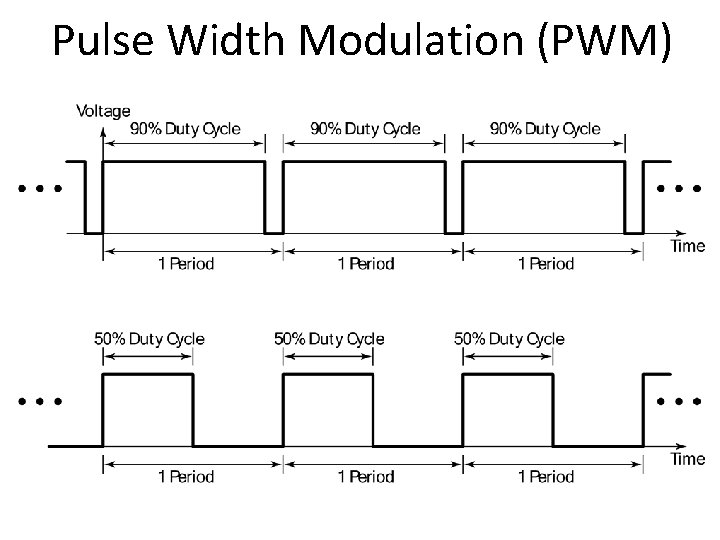Pulse Width Modulation (PWM)Pulse Width Modulation (PWM) 100%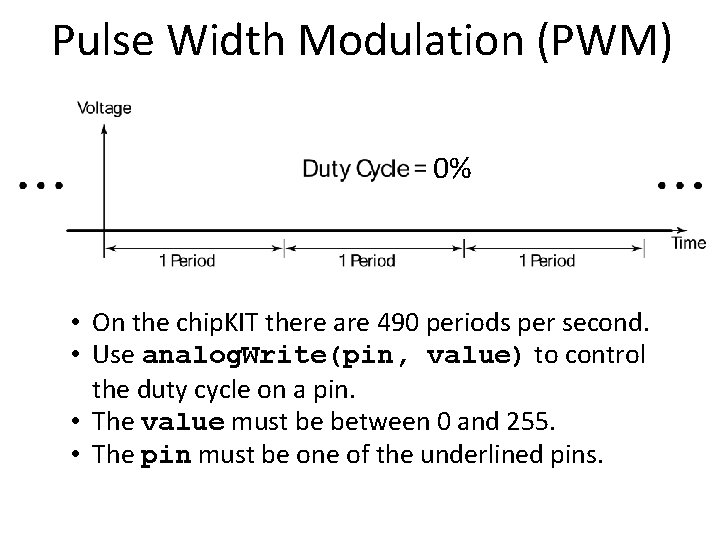Pulse Width Modulation (PWM) 0% • On the chip. KIT there are 490 periods per second. • Use analog. Write(pin, value) to control the duty cycle on a pin. • The value must be between 0 and 255. • The pin must be one of the underlined pins.Pulse Width Modulation (PWM) • If you take the average value over one period, you can think of the voltage as being between 0 V (0% duty cycle) and 3. 3 V (100% duty cycle). • There are 256 different voltage levels (0 to 255).Conversion of Analog to Digital • Obtain “analog” input using analog. Read(). • analog. Read() returns a value between 0 (if pin at ground) and 1023 (if pin at 3. 3 V). • Argument of analog. Read() is the pin to read (only pins labeled ANALOG IN can be used). • Used this to read setting of a potentiometer.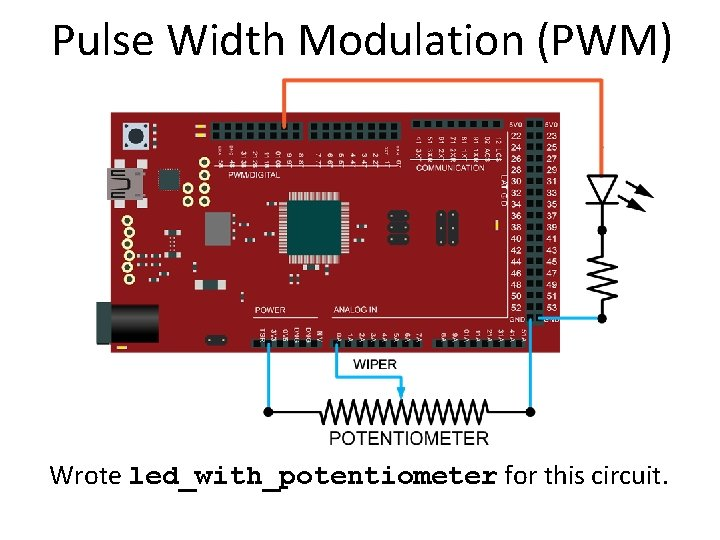Pulse Width Modulation (PWM) Wrote led_with_potentiometer for this circuit.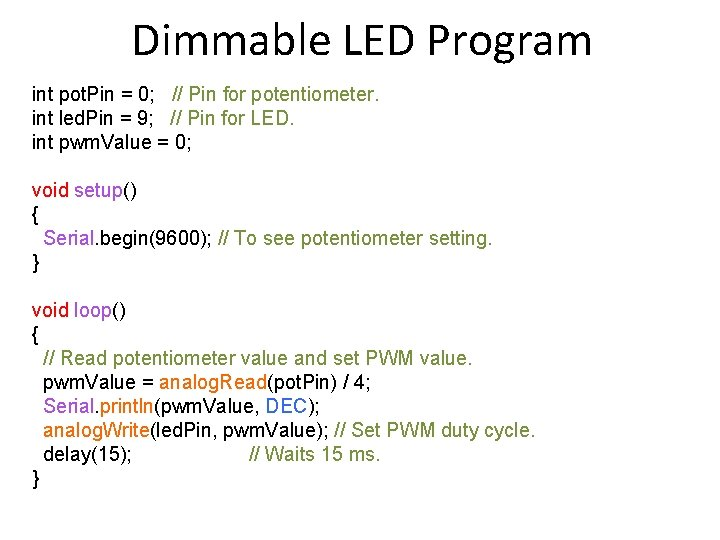Dimmable LED Program int pot. Pin = 0; // Pin for potentiometer. int led. Pin = 9; // Pin for LED. int pwm. Value = 0; void setup() { Serial. begin(9600); // To see potentiometer setting. } void loop() { // Read potentiometer value and set PWM value. pwm. Value = analog. Read(pot. Pin) / 4; Serial. println(pwm. Value, DEC); analog. Write(led. Pin, pwm. Value); // Set PWM duty cycle. delay(15); // Waits 15 ms. }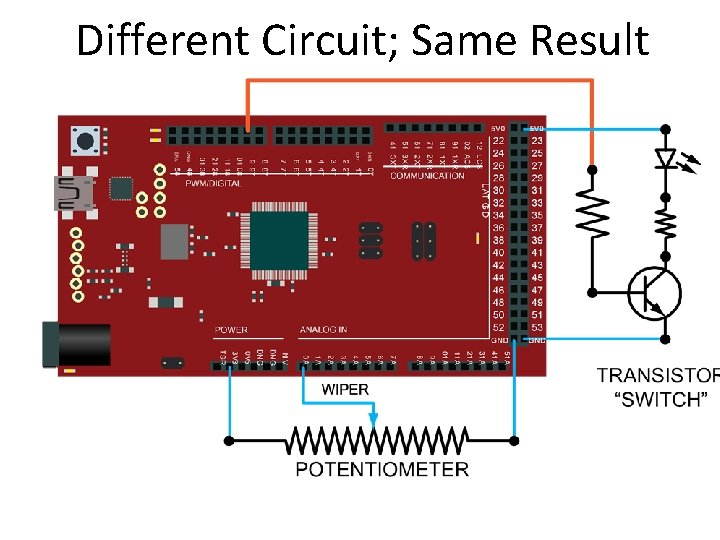Different Circuit; Same Result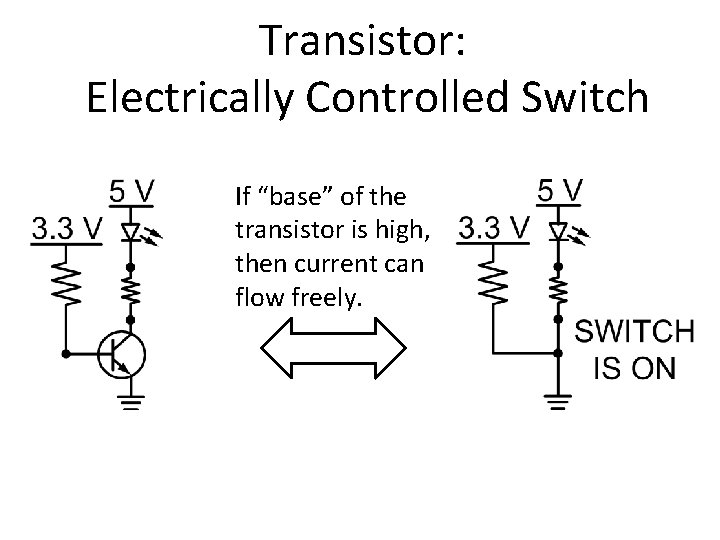Transistor: Electrically Controlled Switch If “base” of the transistor is high, then current can flow freely.Transistor: Electrically Controlled Switch If “base” of the transistor is low, then current cannot flow.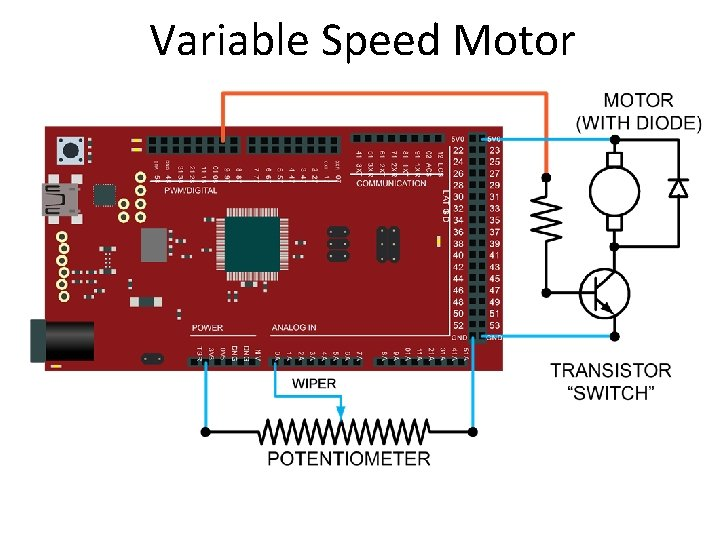Variable Speed Motor